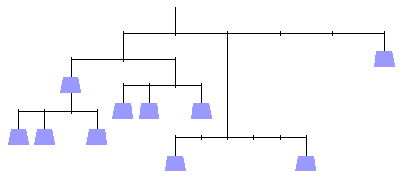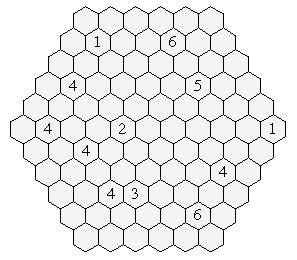1999 U.S. Championship Puzzles

1. Balancing Act

Assign the values 1 to 10 to the weights in the diagram so that everything balances as shown. Each number will be used exactly once.2. Rule of 72

Place the numbers from 1 to 9 in the blanks to make the equation correct. Each digit will be used exactly once. Operations are to be performed strictly from left to right. All intermediate results are positive whole numbers.

__ - __ / __ + __ / __ + __ / __ x __ - __ = 72
3. Hex Aspiration

Find a looped path through the diagram subject to the following constraints. The path proceeds from one cell to an adjacent cell, passes through no cell more than once, does not go through any numbered cells, and never makes a sharp-angled turn (i.e., a turn at a 60o angle). Each number indicates how many of the adjacent cells are part of the path.